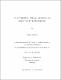## On constrained Markov-Nikolskii and Bernstein type inequalitiesThis thesis is devoted to polynomial inequalities with constraints. We present a history of the development of this subject together with recent progress. In the first part, we solve an analog of classical Markov's problem for monotone polynomials. More precisely, if ∆n denotes the set of all monotone polynomials on [-1,1] of degree n, then for Pn ϵ ∆n and x ϵ [-1,1] the following sharp inequalities hold: │P’n(x)│≤ 2 max(Sk(-x),Sk(-x))║Pn║, for n = 2k + 2, k ≥ 0, and │P'n(x)│ ≤ 2 max (Fk(x), Hk(x))║Pn║, for n = 2k + 1, k ≥ 0, where Sk(x) := (1+x)∑_(l=0 )^k▒(J_l (0,1)(x^2)) ; S_k (x) &:=(1+x)\sum\limits_{l=0}^{k} (J^{(0,1)}_l (x))^2;\\ H_k (x) &:=(1-x^2)\sum\limits_{l=0}^{k-1} (J_l ^{(1,1)} (x))^2;\\ F_k(x) &:=\sum\limits_{l=0}^{k} (J_l ^{(0,0)} (x))^2, \end{align*} and $J_l^{(\alpha,\beta)}(x),$ $l\ge 1$ are the Jacobi polynomials. Let ∆n(1) be the set of all monotone nonnegative polynomials on $[-1,1]$ of degree $n.$ In the second part, we investigate the asymptotic behavior of the constants $$M_{q,p}^{(1)}(n,1):=\sup_{P_n\in\triangle^{(1)}_n}\frac{\|P'_n\|_{L_q [-1,1]}}{\|P_n\|_{L_p [-1,1]}},$$ in constrained Markov-Nikolskii type inequalities. Our conjecture is that $M^{(1)}_{q,p} (n,1)\asymp \left\{ \begin{array}{ll} n^{2+2/p-2/q} , & \mbox{\rm if } 1>1/q-1/p ,\\ \log{n} , & \mbox{\rm if } 1=1/q-1/p, \\ 1 , & \mbox{\rm if } 1< 1/q - 1/p . \end{array} \right.$ We prove this conjecture for all values of p,q > 0, except for the case 0 < q < 1, 1/2 ≤ 1/q- 1/p ≤ 1, p ≠ 1